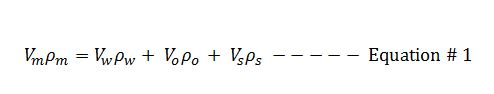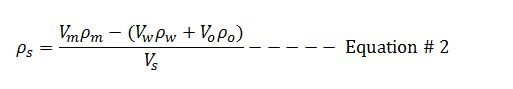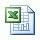## Solid Density From Retort Analysis Mud Calculation

Retort analysis is the method to determine solid and liquid components in the drilling fluid. In this article, we will adapt mass balance and retort analysis data to determine solid density in the mud.Mass balance for mud is listed below;We can rearrange the Euqation#1 in order to determine the solid density## Drilling Formula Excel Sheet Version 1.2 Free Download

This is the second up date of drilling formula spread sheet, Drilling Formula Excel Sheet Version 1.2.

Drillingformulas.com distributed the first version of drilling formula spread sheet on 28 March 2011 and another update on 18 May 2011. The calculation sheet has been downloaded over 8,300 times around the world since the fist distribution.

Today, the lasted up date of drilling formula spread sheet, Drilling Formula Excel Sheet Version 1.2 is available for you to download. It is still totally FREE !!!.What new drilling formulas are added into this version?

Cutting Slip Velocity Method#1

Cutting Slip Velocity Method#2

Surge and Swab Pressure Method#1

Surge and Swab Pressure Method#2

Total Flow Area Table

Calculate Influx Height

Hydrostatic Pressure Loss Due to Gas Cut Mud

Kill Weight Mud

Maximum pit gain from gas kick in water based mud

Maximum Surface Pressure from Gas Influx in Water Based Mud

How many total useful drilling formulas in this version?
There are a total of 75 formulas which are divided into 7 categories (Applied Drilling Formulas, Basic Drilling Formulas, Directional Drilling Calculation, Drilling Fluid Formulas, Engineering Formulas, Hydraulic Formulas and Well Control Formulas).

How much will it cost?

Nothing. The spread sheet is totally FREE for everybody.## Drilling Formula Calculation Sheet Version 1

This is drilling formula calculation sheet v1 distributed on 28-Mar-10. I add many essential drilling formulas in to one Excel spread sheet and I would like to share with you for FREE. Please check this following link for download.

##Drilling Formula Calculation Sheet v1

When you one the file Drilling-formulas-Calculation-shee-V1.xls, you will see the fist page call index page which shows you all of formulas like this.

## Dilution of Mud System to Control Low Gravity Solid by Adding Mud

Adding bbl of  drillingfluid can help control low gravity solid (LGS) in mud system. However, this is different from the way to control LGS by adding base fluid as base oil or water because mud that is added into system has some Low Gravity Solid (LGS). Hence, when we calculate it, we need to account for Low Gravity Solid (LGS) of new mud into the calculation as well. This post will demonstrate you how to determine barrels of drilling fluid required to achive the desired Low Gravity Solid (LGS).

Formula, used to calculate dilution of mud system, is listed below;

Vwm = Vm x (Fct – Fcop) ÷ (Fcop – Fca)

Where; Vwm = barrels of dilution water or base fluid

Vm = total barrels of mud in circulating system

Fct = percent low gravity solids in system

Fcop = percent total low gravity solids desired

Fca = percent low gravity solids bentonite and/or chemicals added in mud

Example: Determine how much barrels of oil base mud to diluate total 2000 bbl of mud in system from total LGS = 7 % to desired LGS of 3.5 %. The oil base mud has 2% of bentonite slurry.

Vwm = Vm x (Fct – Fcop) ÷ (Fcop – Fca)

Vwm = 2000 x (7 – 3.5) ÷ (3.5-2)

Vwm = 4667 bbl

In order to dilute total of 2000 bbl of the original mud with 7% LGS down to 3.5% LGS, 4667 bbl of mud that has 2% bentonite is requied to add into the system.

Please find the excel sheet used to calculate how much barrel of drilling fluid to control Low Gravity Solid (LGS) in mud system.